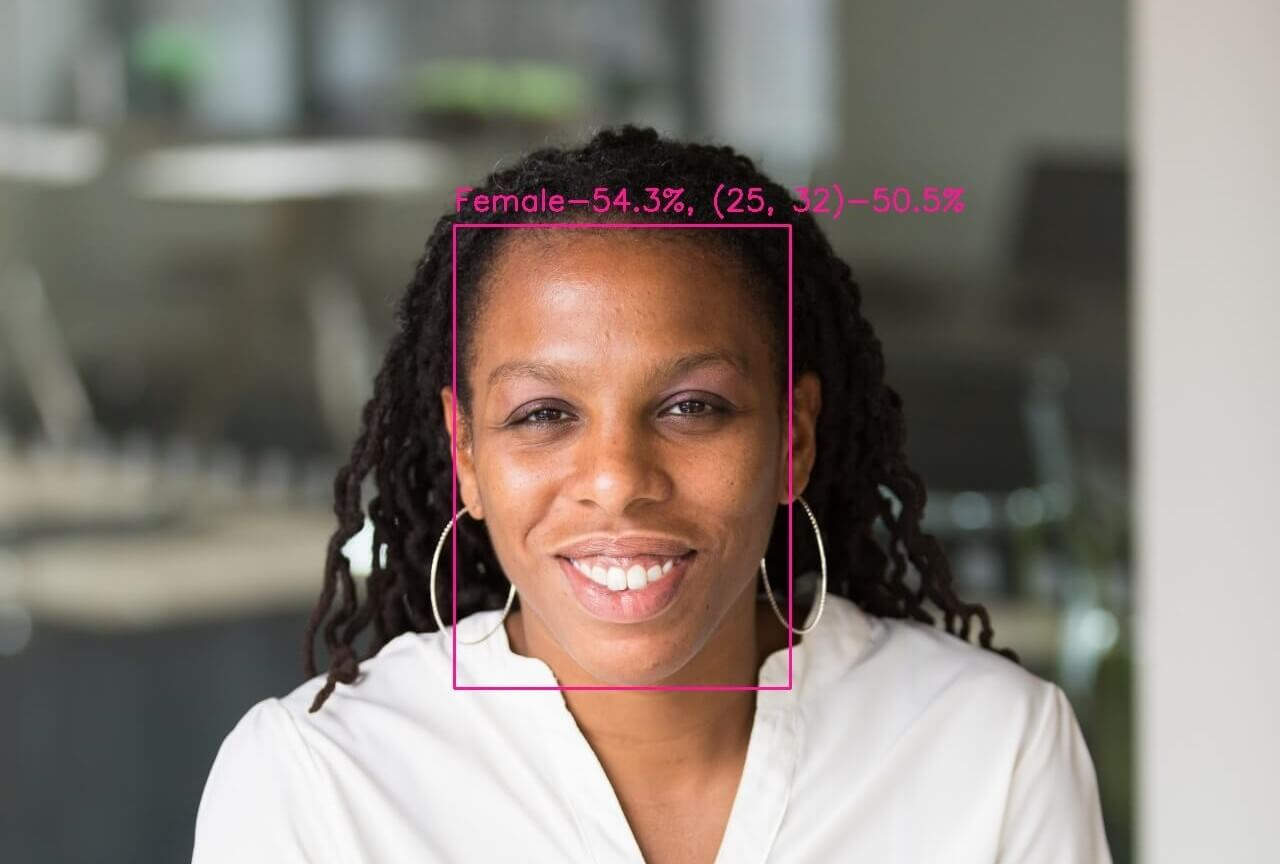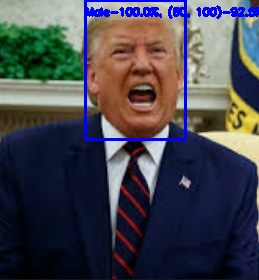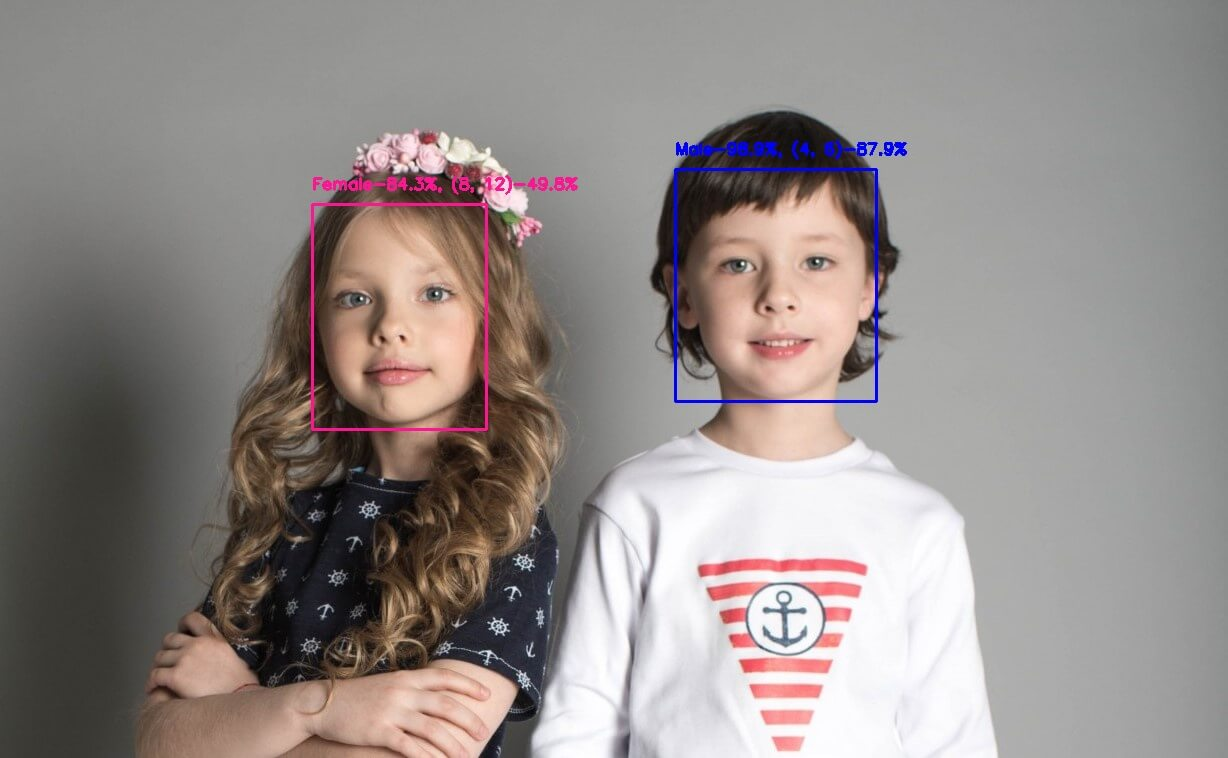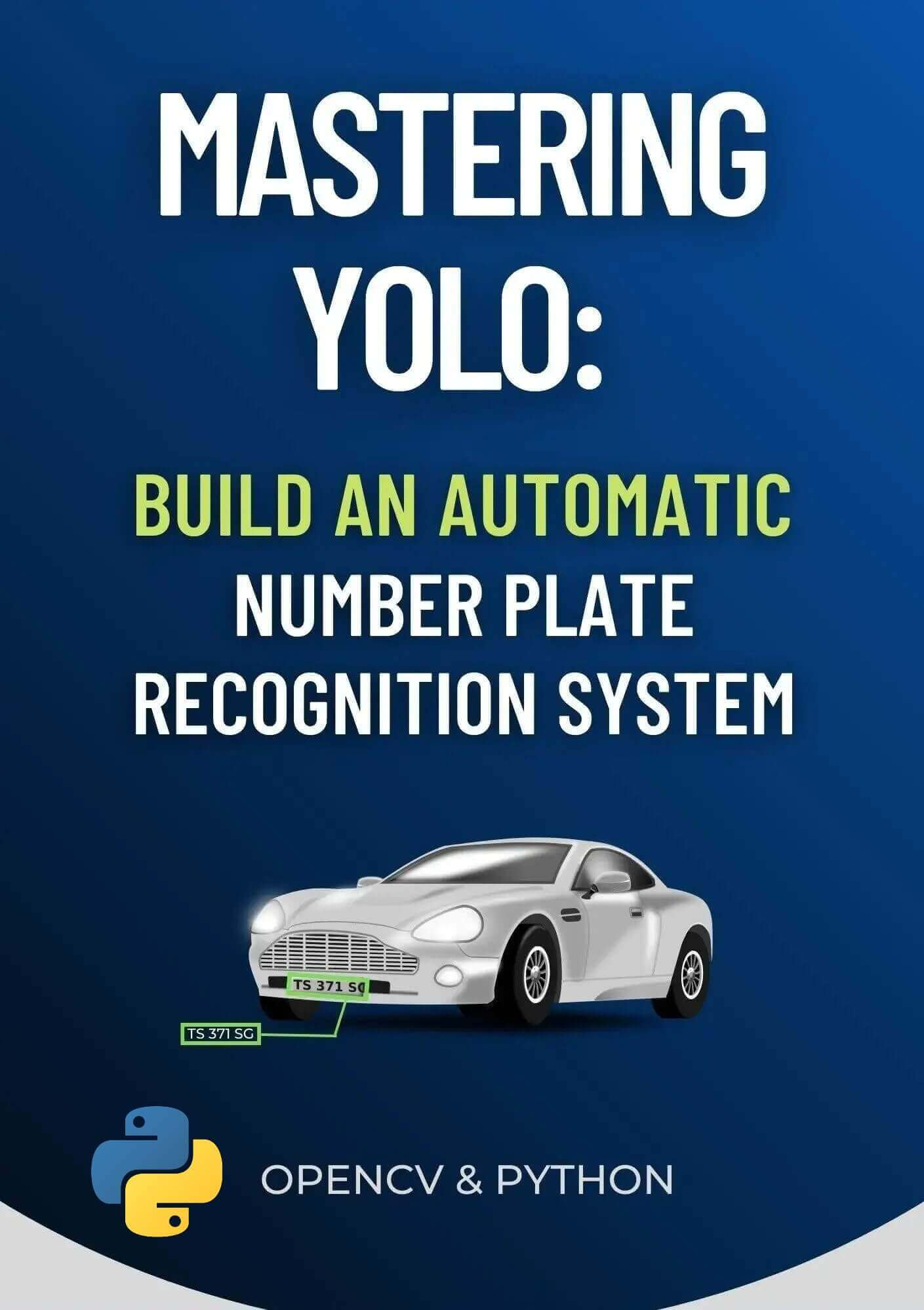# Age and Gender Detection using OpenCV in Python

Learn how to perform age and gender detection using OpenCV library in Python with camera or image input.
7 min read · Updated may 2023 · Machine Learning · Computer Vision

Disclosure: This post may contain affiliate links, meaning when you click the links and make a purchase, we receive a commission.

In this tutorial, we will combine gender detection and age detection tutorials to come up with a single code that detects both.

Let's get started. If you haven't OpenCV already installed, make sure to do so:

``\$ pip install opencv-python numpy``

Open up a new file. Importing the libraries:

``````# Import Libraries
import cv2
import numpy as np``````

Next, defining the variables of weights and architectures for face, age, and gender detection models:

``````# https://raw.githubusercontent.com/opencv/opencv/master/samples/dnn/face_detector/deploy.prototxt
FACE_PROTO = "weights/deploy.prototxt.txt"
# https://raw.githubusercontent.com/opencv/opencv_3rdparty/dnn_samples_face_detector_20180205_fp16/res10_300x300_ssd_iter_140000_fp16.caffemodel
FACE_MODEL = "weights/res10_300x300_ssd_iter_140000_fp16.caffemodel"
# The gender model architecture
GENDER_MODEL = 'weights/deploy_gender.prototxt'
# The gender model pre-trained weights
GENDER_PROTO = 'weights/gender_net.caffemodel'
# Each Caffe Model impose the shape of the input image also image preprocessing is required like mean
# substraction to eliminate the effect of illunination changes
MODEL_MEAN_VALUES = (78.4263377603, 87.7689143744, 114.895847746)
# Represent the gender classes
GENDER_LIST = ['Male', 'Female']
# The model architecture
AGE_MODEL = 'weights/deploy_age.prototxt'
# The model pre-trained weights
AGE_PROTO = 'weights/age_net.caffemodel'
# Represent the 8 age classes of this CNN probability layer
AGE_INTERVALS = ['(0, 2)', '(4, 6)', '(8, 12)', '(15, 20)',
'(25, 32)', '(38, 43)', '(48, 53)', '(60, 100)']``````

Below are the necessary files to be included in the project directory:

• `gender_net.caffemodel`: It is the pre-trained model weights for gender detection. You can download it here.
• `deploy_gender.prototxt`: is the model architecture for the gender detection model (a plain text file with a JSON-like structure containing all the neural network layer’s definitions). Get it here.
• `age_net.caffemodel`: It is the pre-trained model weights for age detection. You can download it here.
• `deploy_age.prototxt`: is the model architecture for the age detection model (a plain text file with a JSON-like structure containing all the neural network layer’s definitions). Get it here.
• `res10_300x300_ssd_iter_140000_fp16.caffemodel`: The pre-trained model weights for face detection, download here.
• `deploy.prototxt.txt`: This is the model architecture for the face detection model, download here.

``````# Initialize frame size
frame_width = 1280
frame_height = 720

Before trying to detect age and gender, we need a function to detect faces first:

``````def get_faces(frame, confidence_threshold=0.5):
# convert the frame into a blob to be ready for NN input
blob = cv2.dnn.blobFromImage(frame, 1.0, (300, 300), (104, 177.0, 123.0))
# set the image as input to the NN
face_net.setInput(blob)
# perform inference and get predictions
output = np.squeeze(face_net.forward())
# initialize the result list
faces = []
# Loop over the faces detected
for i in range(output.shape):
confidence = output[i, 2]
if confidence > confidence_threshold:
box = output[i, 3:7] * \
np.array([frame.shape, frame.shape,
frame.shape, frame.shape])
# convert to integers
start_x, start_y, end_x, end_y = box.astype(np.int)
# widen the box a little
start_x, start_y, end_x, end_y = start_x - \
10, start_y - 10, end_x + 10, end_y + 10
start_x = 0 if start_x < 0 else start_x
start_y = 0 if start_y < 0 else start_y
end_x = 0 if end_x < 0 else end_x
end_y = 0 if end_y < 0 else end_y
# append to our list
faces.append((start_x, start_y, end_x, end_y))
return faces``````

## Mastering YOLO: Build an Automatic Number Plate Recognition System

Building a real-time automatic number plate recognition system using YOLO and OpenCV library in Python

The `get_faces()` function was grabbed from the face detection tutorial, so check it out if you want more information.

Below is a function for simply displaying an image:

``````def display_img(title, img):
"""Displays an image on screen and maintains the output until the user presses a key"""
# Display Image on screen
cv2.imshow(title, img)
# Mantain output until user presses a key
cv2.waitKey(0)
# Destroy windows when user presses a key
cv2.destroyAllWindows()``````

Below are is a function for dynamically resizing an image, we're going to need it to resize the input images when exceeding a certain width:

``````# from: https://stackoverflow.com/questions/44650888/resize-an-image-without-distortion-opencv
def image_resize(image, width = None, height = None, inter = cv2.INTER_AREA):
# initialize the dimensions of the image to be resized and
# grab the image size
dim = None
(h, w) = image.shape[:2]
# if both the width and height are None, then return the
# original image
if width is None and height is None:
return image
# check to see if the width is None
if width is None:
# calculate the ratio of the height and construct the
# dimensions
r = height / float(h)
dim = (int(w * r), height)
# otherwise, the height is None
else:
# calculate the ratio of the width and construct the
# dimensions
r = width / float(w)
dim = (width, int(h * r))
# resize the image
return cv2.resize(image, dim, interpolation = inter)``````

Now that everything is ready, let's define our two functions for age and gender detection:

``````def get_gender_predictions(face_img):
blob = cv2.dnn.blobFromImage(
image=face_img, scalefactor=1.0, size=(227, 227),
mean=MODEL_MEAN_VALUES, swapRB=False, crop=False
)
gender_net.setInput(blob)
return gender_net.forward()

def get_age_predictions(face_img):
blob = cv2.dnn.blobFromImage(
image=face_img, scalefactor=1.0, size=(227, 227),
mean=MODEL_MEAN_VALUES, swapRB=False
)
age_net.setInput(blob)
return age_net.forward()``````

The `get_gender_predictions()` and `get_age_predictions()` perform prediction on the `gender_net` and `age_net` models to infer the gender and age of the input image respectively.

Finally, we write our main function:

``````def predict_age_and_gender(input_path: str):
"""Predict the gender of the faces showing in the image"""
# Initialize frame size
# frame_width = 1280
# frame_height = 720
# resize the image, uncomment if you want to resize the image
# img = cv2.resize(img, (frame_width, frame_height))
# Take a copy of the initial image and resize it
frame = img.copy()
if frame.shape > frame_width:
frame = image_resize(frame, width=frame_width)
# predict the faces
faces = get_faces(frame)
# Loop over the faces detected
# for idx, face in enumerate(faces):
for i, (start_x, start_y, end_x, end_y) in enumerate(faces):
face_img = frame[start_y: end_y, start_x: end_x]
age_preds = get_age_predictions(face_img)
gender_preds = get_gender_predictions(face_img)
i = gender_preds.argmax()
gender = GENDER_LIST[i]
gender_confidence_score = gender_preds[i]
i = age_preds.argmax()
age = AGE_INTERVALS[i]
age_confidence_score = age_preds[i]
# Draw the box
label = f"{gender}-{gender_confidence_score*100:.1f}%, {age}-{age_confidence_score*100:.1f}%"
# label = "{}-{:.2f}%".format(gender, gender_confidence_score*100)
print(label)
yPos = start_y - 15
while yPos < 15:
yPos += 15
box_color = (255, 0, 0) if gender == "Male" else (147, 20, 255)
cv2.rectangle(frame, (start_x, start_y), (end_x, end_y), box_color, 2)
# Label processed image
font_scale = 0.54
cv2.putText(frame, label, (start_x, yPos),
cv2.FONT_HERSHEY_SIMPLEX, font_scale, box_color, 2)

# Display processed image
display_img("Gender Estimator", frame)
# uncomment if you want to save the image
cv2.imwrite("output.jpg", frame)
# Cleanup
cv2.destroyAllWindows()``````

The main function does the following:

• First, it reads the image using the `cv2.imread()` method.
• After the image is resized to the appropriate size, we use our `get_faces()` function to get all the detected faces from the image.
• We iterate on each detected face image and call our `get_age_predictions()` and `get_gender_predictions()` to get the predictions.
• We print the age and gender.
• We draw a rectangle surrounding the face and also put the label that contains the age and gender text along with confidence on the image.
• Finally, we show the image.

Let's call it:

``````if __name__ == "__main__":
import sys
input_path = sys.argv
predict_age_and_gender(input_path)``````

Done, let's run the script now (testing on this image):

``\$ python age_and_gender_detection.py images/girl.jpg``

Output in the console:

``````Male-99.1%, (4, 6)-71.9%
Female-96.0%, (4, 6)-70.9%``````

The resulting image:Here is another example:Or this:Awesome! If you see the text in the image is large or small, make sure to tweak the `font_scale` floating-point variable on your image in the `predict_age_and_gender()` function.

For more detail on how the gender and age prediction works, I suggest you check the individual tutorials:

If you want to use your camera, I made a Python script to read images from your webcam and perform inference in real-time.

Finally, I've collected some useful resources and courses for you for further learning, I highly recommend the following courses:

Check the full code here.

## Mastering YOLO: Build an Automatic Number Plate Recognition System

Building a real-time automatic number plate recognition system using YOLO and OpenCV library in Python

Happy coding ♥

Sharing is caring!

### Comment panel